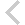FICO Xpress Optimization Examples Repository
 FICO Optimization Community FICO Xpress Optimization HomeFolio - Modelling examples from 'Getting started'

Description
• Chapter 3 Inputting and Solving a Linear Programming problem
• foliolp.mos: modeling and solving a small LP problem
• foliolperr.mos: LP model with syntax errors
• foliolps.mos: LP model using string indices
• Chapter 4 Working with data
• foliodata.mos (data file: folio.dat): data input from file, result output to a file, model parameters
• folioodbc.mos (data files: folio.xls, folio.mdb, folio.sqlite): data input from a spreadsheet or database, result output to a spreadsheet or database, model parameters
• folioexcel.mos (data file: folio.xls): same as folioodbc.mos but with Excel-specific data input and output (Windows only)
• foliosheet.mos (data file: folio.xls): same as folioodbc.mos but with data input and output through generic spreadsheet access
• foliocsv.mos (data file: folio.csv): same as folioodbc.mos but with data input and output through generic spreadsheet access in CSV format
• Chapter 5 Drawing user graphs
• folioloop.mos (data files: folio.dat, foliodev.dat): re-solving with varied parameter settings
• folioloop_graph.mos (data files: folio.dat, foliodev.dat): re-solving with varied parameter settings, graphical solution display
• foliolps_graph.mos: same as foliolps, adding graphical solution display
• Chapter 6 Mixed Integer Programming
• foliomip1.mos (data file: folio.dat): modeling and solving a small MIP problem (binary variables)
• foliomip2.mos (data file: folio.dat): modeling and solving a small MIP problem (semi-continuous variables)
• folioqp.mos (data file: folioqp.dat): modeling and solving a QP and a MIQP problem
• folioqp_graph.mos (data files: folioqp.dat, folioqpgraph.dat): re-solving a QP problem with varied parameter settings, graphical solution display
• folioqc.mos (data file: folioqp.dat): modeling and solving a QCQP and
• foliomiqc.mos (data file: folioqp.dat): modeling and solving a MIQCQP
• Chapter 8 Heuristics
• folioheur.mos (data file: folio.dat): heuristic solution of a MIP problem

Source Files

Data Files

foliolp.mos

(!******************************************************
Mosel Example Problems
======================

file foliolp.mos

Modeling a small LP problem
to perform portfolio optimization.

(c) 2008 Fair Isaac Corporation
author: S.Heipcke, Aug. 2003
*******************************************************!)

model "Portfolio optimization with LP"
uses "mmxprs"

declarations
SHARES = 1..10                     ! Set of shares
RISK = {2,3,4,9,10}                ! Set of high-risk values among shares
NA = {1,2,3,4}                     ! Set of shares issued in N.-America
RET: array(SHARES) of real         ! Estimated return in investment

frac: array(SHARES) of mpvar       ! Fraction of capital used per share
end-declarations

RET:: [5,17,26,12,8,9,7,6,31,21]

! Objective: total return
Return:= sum(s in SHARES) RET(s)*frac(s)

! Limit the percentage of high-risk values
sum(s in RISK) frac(s) <= 1/3

! Minimum amount of North-American values
sum(s in NA) frac(s) >= 0.5

! Spend all the capital
sum(s in SHARES) frac(s) = 1

! Upper bounds on the investment per share
forall(s in SHARES) frac(s) <= 0.3

! Solve the problem
maximize(Return)

(!
declarations
Status:array({XPRS_OPT,XPRS_UNF,XPRS_INF,XPRS_UNB,XPRS_OTH}) of string
end-declarations

Status::([XPRS_OPT,XPRS_UNF,XPRS_INF,XPRS_UNB,XPRS_OTH])[
"Optimum found","Unfinished","Infeasible","Unbounded","Failed"]

writeln("Problem status: ", Status(getprobstat))
!)

! Solution printing
writeln("Total return: ", getobjval)
forall(s in SHARES) writeln(s, ": ", getsol(frac(s))*100, "%")

end-model